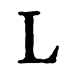Tämä sivusto käyttää evästeitä palvelujen toimittamiseen, toiminnan parantamiseen, analytiikkaan ja (jos et ole kirjautunut sisään) mainostamiseen. Käyttämällä LibraryThingiä ilmaiset, että olet lukenut ja ymmärtänyt käyttöehdot ja yksityisyydensuojakäytännöt. Sivujen ja palveluiden käytön tulee olla näiden ehtojen ja käytäntöjen mukaista.
Hide this

### Tulokset Google Booksista

Pikkukuvaa napsauttamalla pääset Google Booksiin.

Ladataan...

# CRC Standard Mathematical Tables (vuoden 1970 painos)

 Jäseniä Kirja-arvosteluja Suosituimmuussija Keskimääräinen arvio Maininnat 720 6 23,087(3.92) 5 The 30th edition still provides you with all of the usual tools. As it always has, the handbook covers numbers, geometry, trigonometry, calculus, special functions, numerical methods, probability, and statistics. This is an excellent reference handbook for modern mathematics, filled with tables, formulae, equations, and descriptions.… (lisätietoja)
 Jäsen: wehrwulf Teoksen nimi: CRC Standard Mathematical Tables Kirjailijat: Info: The Chemical Rubber Com., Cleveland Kokoelmat: Oma kirjasto Arvio (tähdet):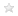Avainsanoja: -

## Teoksen tarkat tiedot

CRC standard mathematical tables (tekijä: William H. Beyer (Editor))

 Viimeisimmät tallentajat Baphijmm, ChanningGauruder, byuecon, Farragut, leslie2894, unkilbeeg, genepeters, pszolovits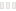Ladataan...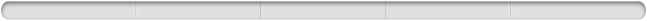Kirjaudu LibraryThingiin, niin näet, pidätkö tästä kirjasta vai et.

Ei tämänhetkisiä Keskustelu-viestiketjuja tästä kirjasta.

» Katso myös 5 mainintaa

englanti (5)  tanska (1)  Kaikki kielet (6)
Näyttää 1-5 (yhteensä 6) (seuraava | näytä kaikki)
NAIndeholder "Preface", "Greek Alphabet", "The Number of Each Day of the Year", "I. CONSTANTS AND CONVERSION FACTORS", " SI System of Measurement", " Conversion Factors", " Metric to English / English to Metric", " Temperature Factors", " Decimal Equivalents to Fractions of an Inch", " Physical Constants", " Miscellaneous Math Constants/Numbers Containing pi, e", "II. ALGEBRA", " Factors and Expansions, Powers and Roots, Proportions, Progressions, Solutions of Equations, Partial Fractions", " Algebra of Sets", " Matrices and Determinants", "III. COMBINATORIAL ANALYSIS", " Squares, Square Root, Cubes and Cube Root", " Powers of Numbers", " Positive Powers of Two", " Negative Powers of Two", " Sums of Powers of Integers", " Sums of Reciprocal Powers of Integers", " Factorials, Factorials and their Logarithms, Reciprocals of Factorials and their Logarithms", " Permutations P(n, m)", " Combinations (n over m) = C(n, m)", " Properties of Binomial Coefficients", " Primes - 1 to 100,000", " Factors and Primes", " Totient Function", " Indices and Power Residues", " Primitive Roots for Primes 3 to 5003", " Positional Notation/change of Base", " Binary and Octal Scales", " Octal-Decimal Conversion", " Hexadecimal and Decimal Conversion", "IV. GEOMETRY", " Mensuration Formulas: Plane Figures and Solids", " Reciprocals, Circumference, and Areas of Circles", "V. TRIGONOMETRY", " Formulas for Use in Trigonometry", " Formulas for Use in Spherical Trigonometry", " Degrees-Radians, Radians-Degrees Convertion Tables", " Natural Trigonometric Functions to Five Places", " Natural Trigonometric Functions for Angles in Radians", " Natural Trigonometric Functions - Secants and Cosecants for Angles in Radians", " Natural Trigonometric Functions - Sine, Tangent, Cotangent, Cosine for Angles in pi * x Radians", " Sine and Cosine Functions for Spial Multiples of pi Radians", "VI. LOGARITHMIC, EXPONENTIAL, AND HYPERBOLIC FUNCTIONS", " Laws of Exponents and Logarithms", " Five-place Mantissas for Common Logarithms", " Natural or Naperian Logarithms", " Exponential Functions", " Hyperbolic Functíons: Formulas", " Inverse Hyperbolic Functíons: Formulas", " Gudermannian Function", " Hyperbolic Functíons and Their Common Logarithms", " Exponential and Hyperbolic Functions for pi * x", " Inverse Hyperbolic Functíons", " Gudermannian Function", "VII. ANALYTIC GEOMETRY", " Formulas for Use in Analytic Geometry", " Rectangular Coordinales in a Plane", " Oblique Coordinates in a Plane", " Polar Coordinates in a Plane", " Rectangular Coordinates in Space", " Cylindrical and Spherical Coordinates", " Curves and Surfaces", " Plane Curves", " Quadric Surfaces", "VIII. CALCULUS", " Derivatives", " Integration", " Elementary Forms", " Definite Integrals", " Series Expansions: Binomial, Reversion of Series, Taylor, Maclaurin, Exponential, Logarithmic, Trigonometric", " Vector Analysis", " Moment Mass of Inertia for Various Bodies of", "IX. DIFFERENTIAL EQUATIONS", " Methods of Solution: First Order First Degree Equations, Linear Equations with Constant Coefficients, Cauchy Equation, Bessel's Equation, Legendre's Equation", " Special Formulas: nth Order Differential Equations with Constant Coefficients", "X. SPECIAL FUNCTIONS", " Gamma Function", " Beta Function", " Error Function", " Bessel Functions", " Bessel Functions Jo(x) and Ji(x)", " Bessel Functions for Spherical Coordinates", " Hyperbolic Bessel Functions", " Elliptic Integrals of the First, Second, and Third Kind", " Elliptic Integrals of the First Kind", " Elliptic Integrals of the Second Kind", " Complete Elliptic Integrals", " Sine, Cosine, and Exponential Integrals", " Orthogonal Polynomials - Legendre, Tschebysheff, Jacobi, Laguerre, Hermite", " Surface Zonal Harmonics", " Bernoulli and Euler Numbers - Polynomials", " Cooefficients of Bernoulli and Euler Polynomials", " Bernoulli Numbers", " Euler Numbers", " Bernoulli and Euler Polynomials", " Stirling Numbers", " Fourier Series", " Fourier Expansions for Basic Periodic Functions", " Fourier Transforms", " Laplace Transform", " The Z-Transform", "XI. NUMERICAL METHODS", " Finite Differences", " Interpolation Formulas", " Differentiation Formulas", " Integration Formulas", "XII. PROBABILITY AND STATISTICS", " Descriptive Statistics: Definitions and Formulas", " Probability", " Probability Distributions", " Normal Probability Function", " Cumulative Terms, Binomial Distribution", " Cumulative Terms, Poisson Distribution", " Percentage Points, Student's t-Distribution", " Percentage Points, Chi-Square Distribution", " Percentage Points, F-Distribution", " Random Units", "XIII. FINANCIAL TABLES", " Formulas for Interest Tables", " Amount at Compound Intererest (1+r)^n", " Present Value 1/(1+i)^n", " Compound Amount of 1 for Fractional Periods (1+i)^(1/p)", " Commissioners 1941 and 1958 Standard Ordinary Mortality Table", "Index".

Glimrende samling af formler og tal. Jeg har brugt mange timer på at løse fjerdegradsligninger vha formlen i denne bog. Der er dog vældig mange trykfejl i indholdsfortegnelsen, så jeg tror de har haft en praktikant til at lave den. ()I purchased this book used for much less that \$1.00 (maybe 10 cents) in 1970 or 71 for the log tables and formulas and used it in my classes at Laney College in Oakland, CA. (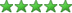)A great old book of tables for when we actually used logarithms for calculation. Electricity! Who needs electricity I still have my trusty slide rule. :-) (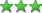)You can always tell the age of the student by the edition of the CRC. It is one of my favorite books. No it's not a turn paging novel or an inspiring or riveting nonfiction work, but it does provide me with a wealth of knowledge. It has been a good friend these many years. ()Näyttää 1-5 (yhteensä 6) (seuraava | näytä kaikki)
Sinun täytyy kirjautua sisään voidaksesi muokata Yhteistä tietoa
Katso lisäohjeita Common Knowledge -sivuilta (englanniksi).
Kanoninen teoksen nimiAlkuteoksen nimiTeoksen muut nimetAlkuperäinen julkaisuvuosiHenkilöt/hahmotTärkeät paikatTärkeät tapahtumatKirjaan liittyvät elokuvatPalkinnot ja kunnianosoituksetEpigrafi (motto tai mietelause kirjan alussa)OmistuskirjoitusEnsimmäiset sanatSitaatitViimeiset sanatErotteluhuomautusJulkaisutoimittajatKirjan kehujatAlkuteoksen kieliCanonical DDC/MDSViittaukset tähän teokseen muissa lähteissä.

##Englanninkielinen Wikipedia

-

The 30th edition still provides you with all of the usual tools. As it always has, the handbook covers numbers, geometry, trigonometry, calculus, special functions, numerical methods, probability, and statistics. This is an excellent reference handbook for modern mathematics, filled with tables, formulae, equations, and descriptions.

No library descriptions found.

Kirjan kuvailuYhteenveto haiku-muodossa## Arvio (tähdet)

Keskiarvo:(3.92)
 0.5 11 1.5 22 2.5 37 3.52 46 4.51 512

## Oletko sinä tämä henkilö?

Lisätietoja | Ota yhteyttä | LibraryThing.com | Yksityisyyden suoja / Käyttöehdot | Apua/FAQ | Blogi | Kauppa | APIs | TinyCat | Perintökirjastot | Varhaiset kirja-arvostelijat | Yleistieto | 157,012,220 kirjaa! | Yläpalkki: Aina näkyvissä